SNVS089O July   2000  – July 2015

PRODUCTION DATA.

1. Features
2. Applications
3. Description
4. Revision History
5. Pin Configuration and Functions
6. Specifications
7. Detailed Description
8. Application and Implementation
1. 8.1 Application Information
2. 8.2 Typical Applications
1. 8.2.1 Boost Converter
2. 8.2.2 Designing SEPIC Using LM3488
1. 8.2.2.1 Design Requirements
2. 8.2.2.2 Detailed Design Procedure
9. Power Supply Recommendations
10. 10Layout
11. 11Device and Documentation Support
12. 12Mechanical, Packaging, and Orderable Information

• DGK|8
• DGK|8

## 7 Detailed Description

### 7.1 Overview

The LM3488 uses a fixed frequency, Pulse Width Modulated (PWM), current mode control architecture. In a typical application circuit, the peak current through the external MOSFET is sensed through an external sense resistor. The voltage across this resistor is fed into the ISEN pin. This voltage is then level shifted and fed into the positive input of the PWM comparator. The output voltage is also sensed through an external feedback resistor divider network and fed into the error amplifier negative input (feedback pin, FB). The output of the error amplifier (COMP pin) is added to the slope compensation ramp and fed into the negative input of the PWM comparator.

At the start of any switching cycle, the oscillator sets the RS latch using the SET/Blank-out and switch logic blocks. This forces a high signal on the DR pin (gate of the external MOSFET) and the external MOSFET turns on. When the voltage on the positive input of the PWM comparator exceeds the negative input, the RS latch is reset and the external MOSFET turns off.

The voltage sensed across the sense resistor generally contains spurious noise spikes, as shown in Figure 20. These spikes can force the PWM comparator to reset the RS latch prematurely. To prevent these spikes from resetting the latch, a blank-out circuit inside the IC prevents the PWM comparator from resetting the latch for a short duration after the latch is set. This duration is about 150ns and is called the blank-out time.

Under extremely light load or no-load conditions, the energy delivered to the output capacitor when the external MOSFET is on during the blank-out time is more than what is delivered to the load. An over-voltage comparator inside the LM3488 prevents the output voltage from rising under these conditions. The over-voltage comparator senses the feedback (FB pin) voltage and resets the RS latch under these conditions. The latch remains in reset state till the output decays to the nominal value.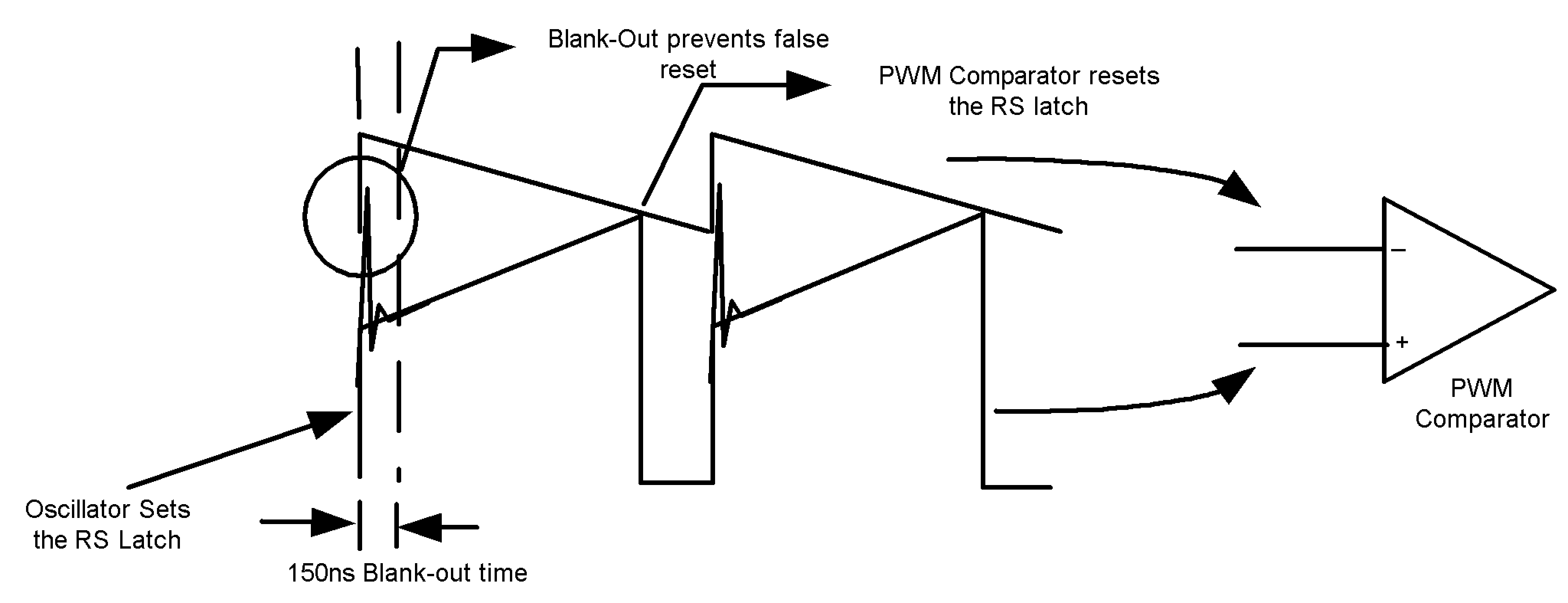Figure 20. Basic Operation of the PWM Comparator

### 7.2 Functional Block Diagram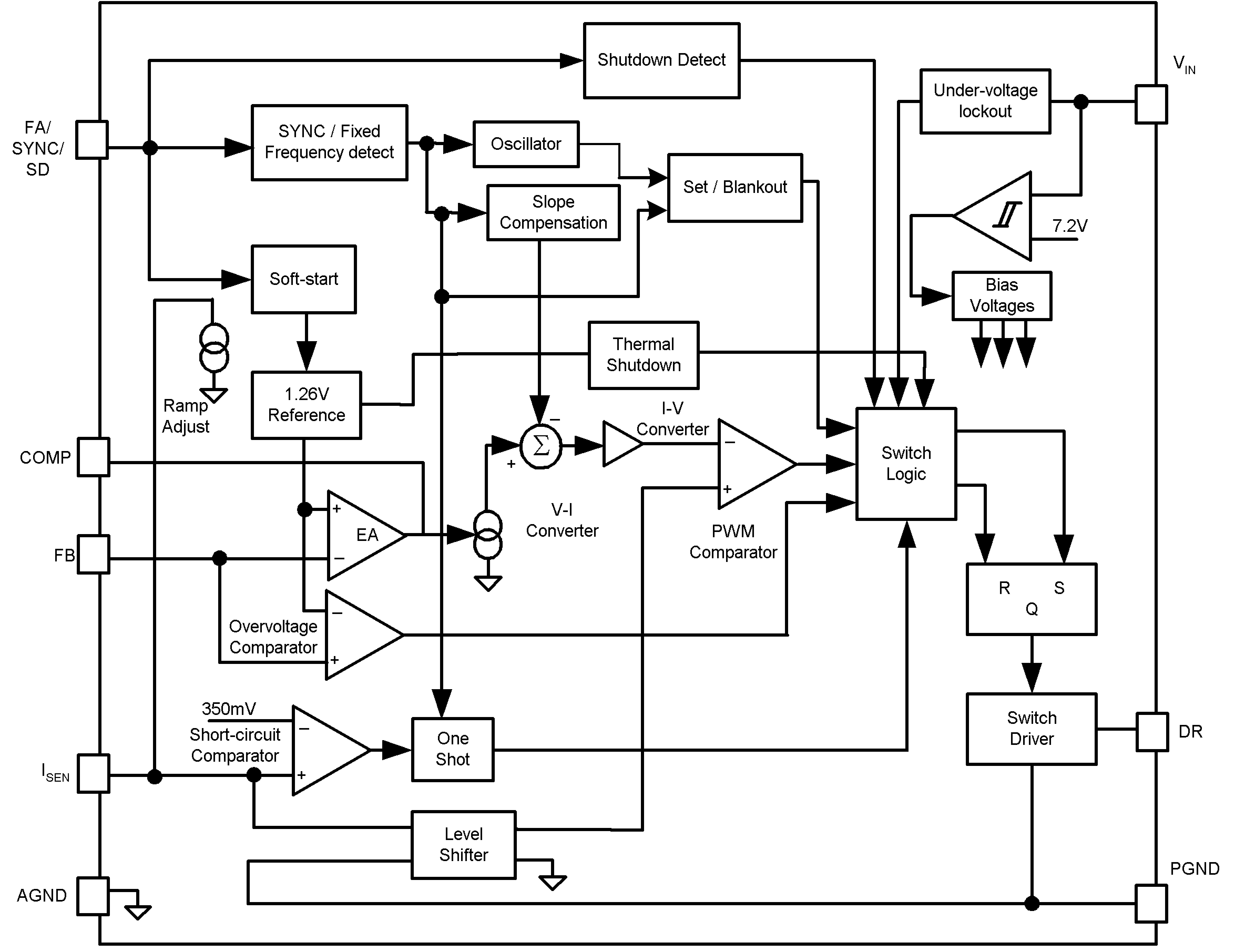### 7.3 Feature Description

#### 7.3.1 Slope Compensation Ramp

The LM3488 uses a current mode control scheme. The main advantages of current mode control are inherent cycle-by-cycle current limit for the switch, and simpler control loop characteristics. It is also easy to parallel power stages using current mode control since as current sharing is automatic.

Current mode control has an inherent instability for duty cycles greater than 50%, as shown in Figure 21. In Figure 21, a small increase in the load current causes the switch current to increase by ΔIO. The effect of this load change, ΔI1, is :

Equation 1.From the above equation, when D > 0.5, ΔI1 will be greater than ΔIO. In other words, the disturbance is divergent. So a very small perturbation in the load will cause the disturbance to increase.

To prevent the sub-harmonic oscillations, a compensation ramp is added to the control signal, as shown in Figure 22.

With the compensation ramp,

Equation 2.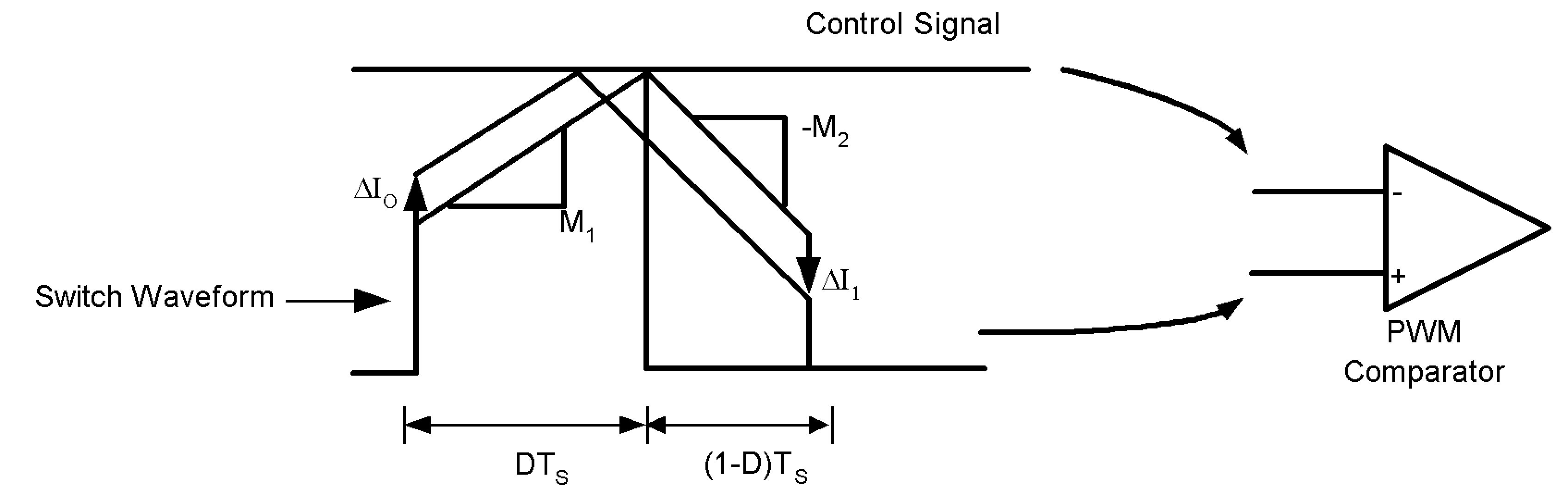Figure 21. Sub-Harmonic Oscillation for D>0.5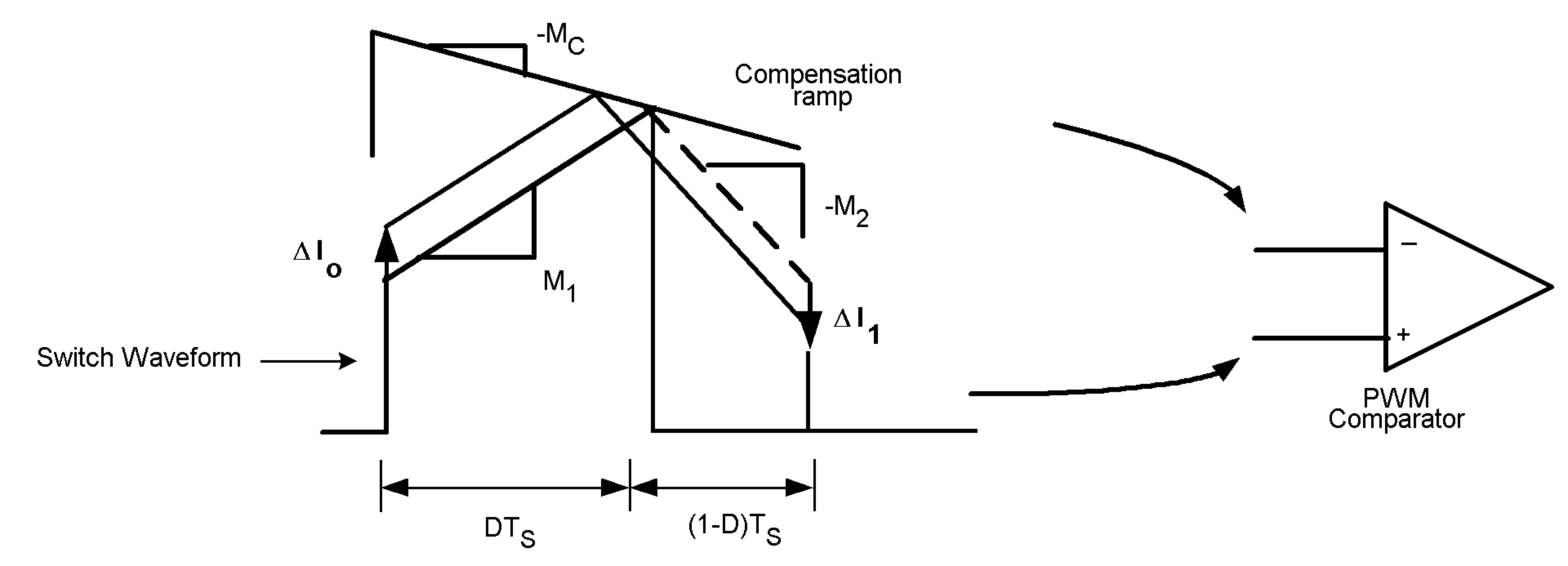Figure 22. Compensation Ramp Avoids Sub-Harmonic Oscillation

The compensation ramp has been added internally in LM3488. The slope of this compensation ramp has been selected to satisfy most of the applications. The slope of the internal compensation ramp depends on the frequency. This slope can be calculated using the formula:

Equation 3. MC = VSL.FS Volts/second

In the above equation, VSL is the amplitude of the internal compensation ramp. Limits for VSL have been specified in the electrical characteristics.

In order to provide the user additional flexibility, a patented scheme has been implemented inside the IC to increase the slope of the compensation ramp externally, if the need arises. Adding a single external resistor, RSL(as shown in Figure 23) increases the slope of the compensation ramp, MC by :

Equation 4.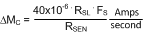In this equation, ΔVSL is equal to 40.10-6RSL. Hence,

Equation 5.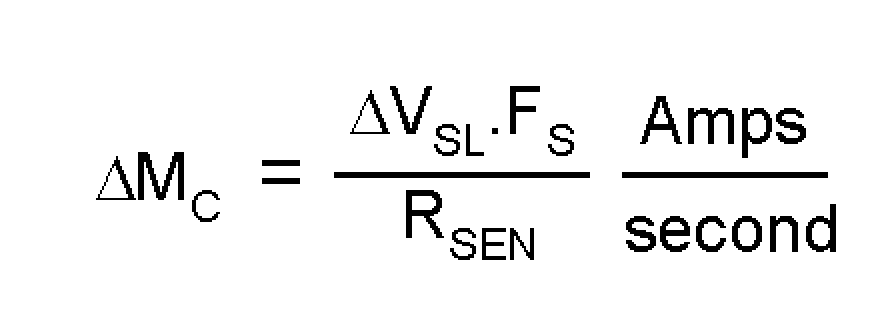ΔVSL versus RSL has been plotted in Figure 24 for different frequencies.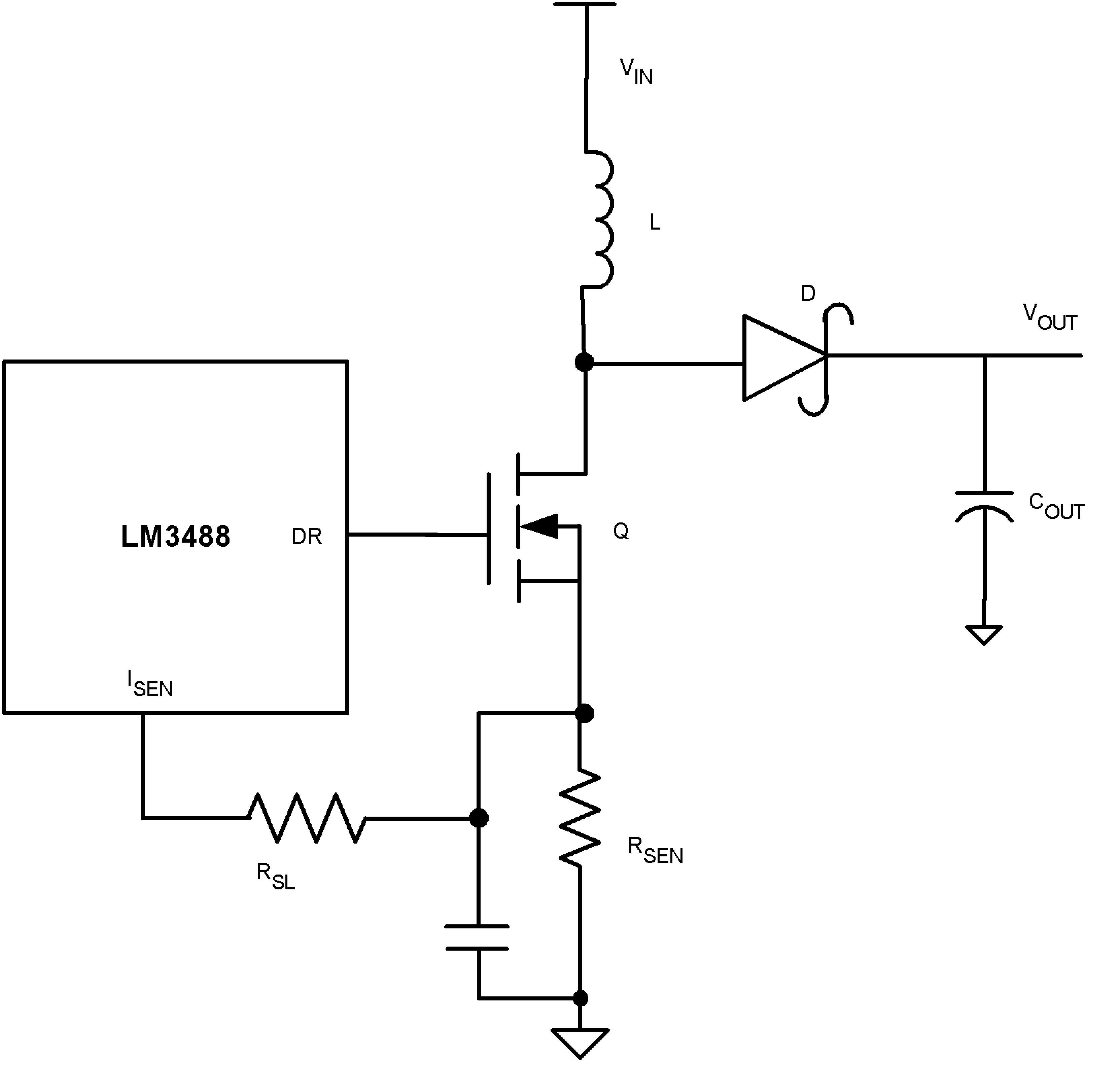Figure 23. Increasing the Slope of the Compensation Ramp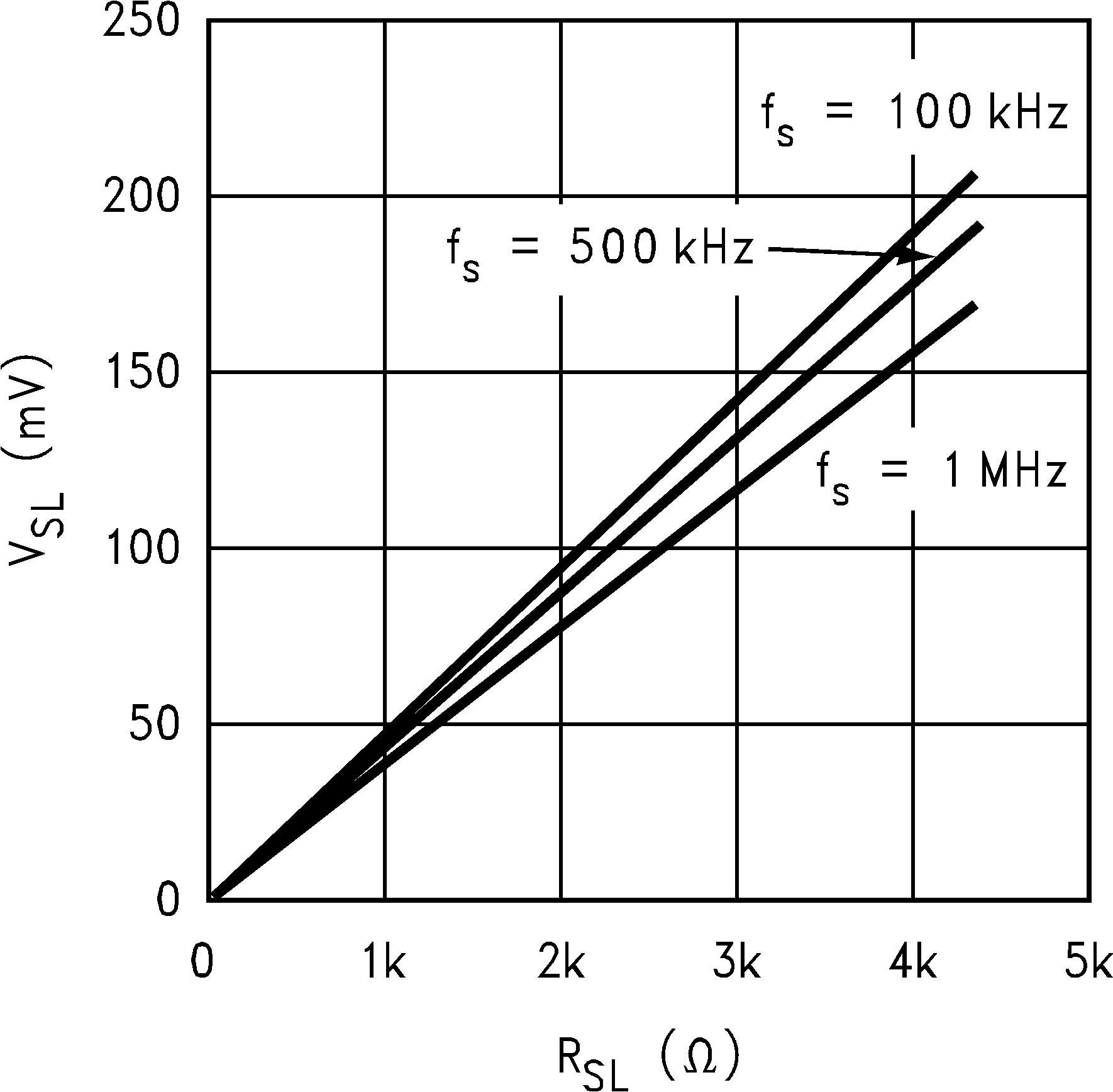Figure 24. ΔVSL vs RSL

The switching frequency of LM3488 can be adjusted between 100kHz and 1MHz using a single external resistor. This resistor must be connected between FA/SYNC/SD pin and ground, as shown in Figure 25. See Typical Characteristics to determine the value of the resistor required for a desired switching frequency.

The LM3488 can be synchronized to an external clock. The external clock must be connected to the FA/SYNC/SD pin through a resistor, RSYNC as shown in Figure 26. The value of this resistor is dependent on the off time of the synchronization pulse, TOFF(SYNC). Table 1 shows the range of resistors to be used for a given TOFF(SYNC).

### Table 1. Recommended Series Resistance for Synchronization

TOFF(SYNC) (µs) RSYNC range (kΩ)
1 5 to 13
2 20 to 40
3 40 to 65
4 55 to 90
5 70 to 110
6 85 to 140
7 100 to 160
8 120 to 190
9 135 to 215
10 150 to 240

It is also necessary to have the width of the synchronization pulse wider than the duty cycle of the converter (when DR pin is high and the switching point is low). It is also necessary to have the synchronization pulse width ≥ 300nsecs.

The FA/SYNC/SD pin also functions as a shutdown pin. If a high signal (see Electrical Characteristics for definition of high signal) appears on the FA/SYNC/SD pin, the LM3488 stops switching and goes into a low current mode. The total supply current of the IC reduces to less than 10µA under these conditions.

Figure 27 and Figure 28 show implementation of shutdown function when operating in Frequency adjust mode and synchronization mode respectively. In frequency adjust mode, connecting the FA/SYNC/SD pin to ground forces the clock to run at a certain frequency. Pulling this pin high shuts down the IC. In frequency adjust or synchronization mode, a high signal for more than 30µs shuts down the IC.

Figure 29 shows implementation of both frequency adjust with RFA resistor and frequency synchronization with RSYNC. The switching frequency is defined by RFA when a synchronization signal is not applied. When sync is applied it overrides the RFA setting.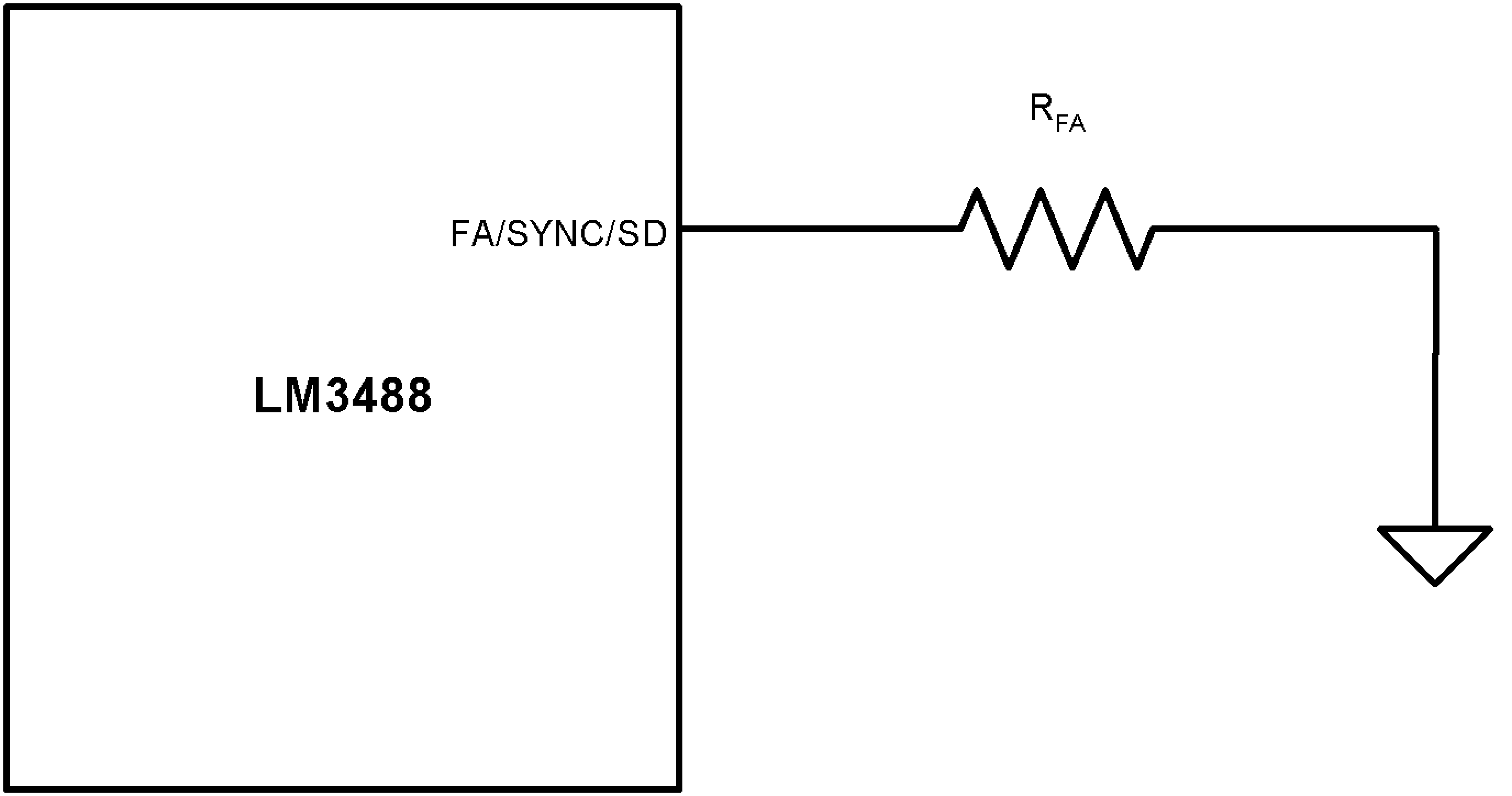Figure 25. Frequency Adjust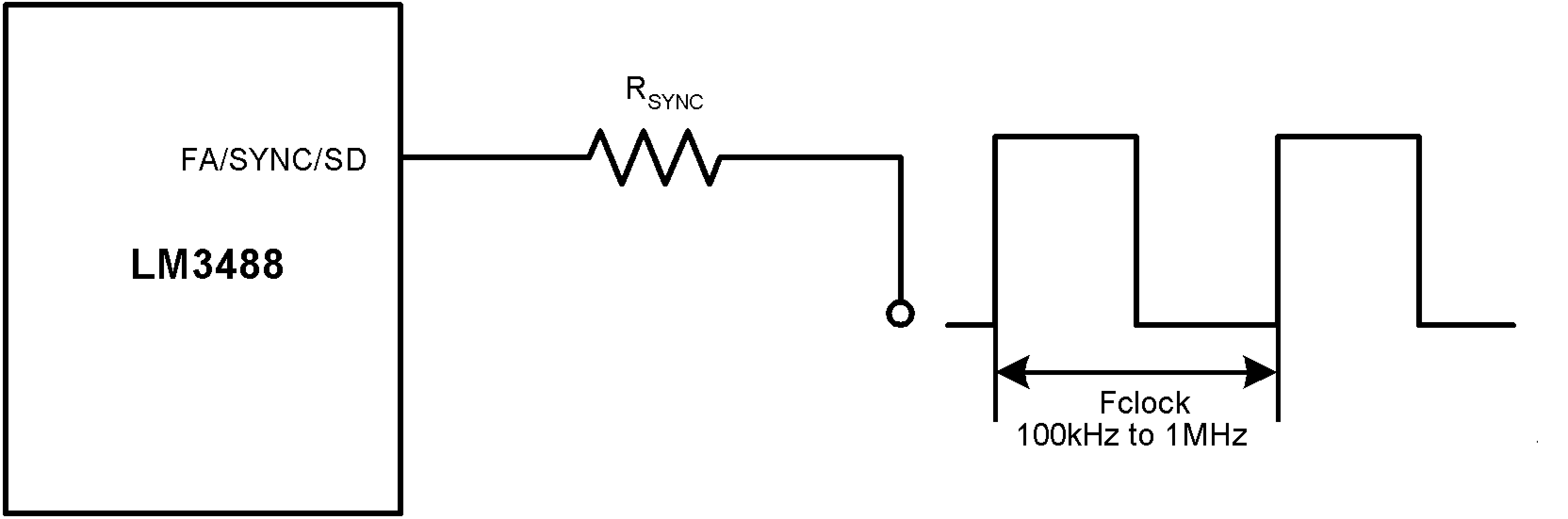Figure 26. Frequency Synchronization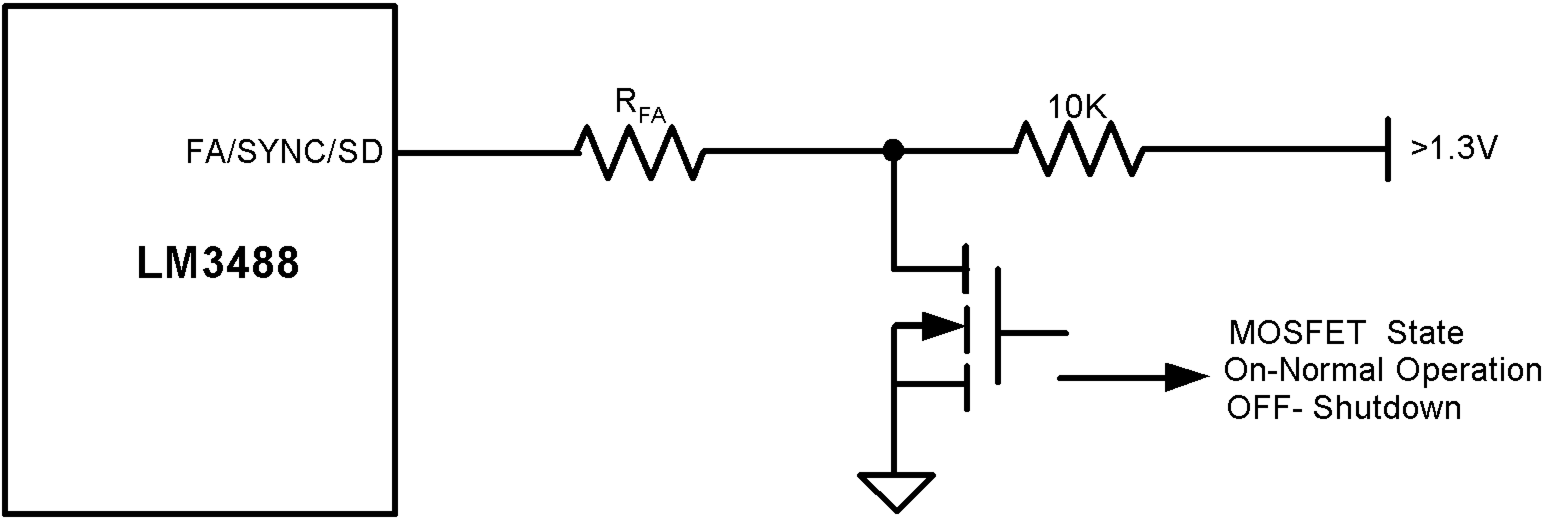Figure 27. Shutdown Operation in Frequency Adjust Mode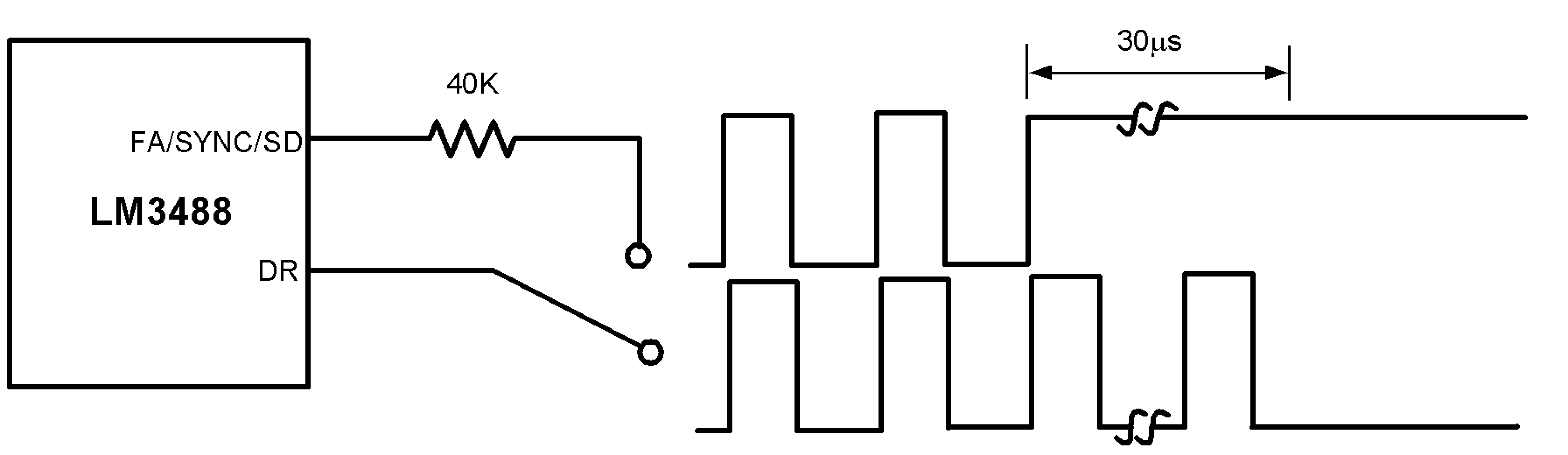Figure 28. Shutdown Operation in Synchronization Mode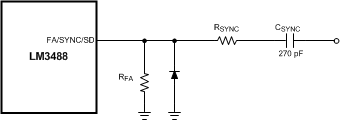Figure 29. Frequency Adjust or Frequency Synchronization

#### 7.3.3 Short-Circuit Protection

When the voltage across the sense resistor (measured on ISEN Pin) exceeds 350mV, short-circuit current limit gets activated. A comparator inside LM3488 reduces the switching frequency by a factor of 5 and maintains this condition till the short is removed.

### 7.4 Device Functional Modes

The device is set to run as soon as the input voltage crosses above the UVLO set point and at a frequency set according to the FA/SYNC/SD pin pull-down resistor or to run at a frequency set by the waveform applied to the FA/SYNC/SD pin.

If the FA/SYNC/SD pin is pulled high, the LM3488 enters shut-down mode.

If the voltage at the ISEN pin exceeds Vsc, the device enters short-circuit protection mode.